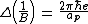# De Haas–van Alphen effect

## De Haas–van Alphen effect

An oscillatory behavior of the magnetic moment of a pure metal crystal with changes in the applied magnetic field B, at very low temperatures. It is named after its discoverers, W. J. de Haas and P. M. van Alphen. This effect has its origin in the Bohr-Sommerfeld quantization of the orbits of conduction electrons under the influence of the magnetic field.

A measurement of the temperature dependence of the oscillation amplitude permits a determination of the cyclotron frequency, or equivalently the electron mass. In a metal, as in a semiconductor, electrons behave with an effective mass m* rather than the free electron mass, and the de Haas–van Alphen effect thus allows a measurement of m* in metals. See Kinetic theory of matter

Application of the Bohr-Sommerfeld quantization rules shows that the “period” of the de Haas–van Alphen oscillations (it is a period in magnetic field, not time) is given by the equation below. Here ap is the area of the orbit in momentumspace of an electron at the Fermi level, that is, the cross section of the Fermi surface; e is the charge of the electron; and &hstrok; is Planck's constant divided by 2π. By studying the dependence of ap on the direction B of the magnetic field, sufficient information can be obtained to construct the detailed shape of the Fermi surfaces of a metal.

Virtually all metals available in sufficient purity have been studied by the de Haas–van Alphen effect. The effect is also the most powerful probe of Fermi surface properties in alloys and intermetallic compounds. See Band theory of solids, Fermi surface

McGraw-Hill Concise Encyclopedia of Physics. © 2002 by The McGraw-Hill Companies, Inc.
Site: Follow: Share:
Open / Close